I've written about how to generate a sample from a multivariate normal (MVN) distribution in SAS by using the RANDNORMAL function in SAS/IML software. Last week a SAS/IML programmer showed me a program that simulated MVN data and computed the resulting covariance matrix for each simulated sample. The purpose of the program was to study properties of the covariance matrices.

The programmer was pleased when I told him that SAS/IML software provides a simpler and more efficient way to simulate covariance and correlation matrices for MVN data. You can generate the covariance matrices directly by using the RANDWISHART function, which generates matrices from the Wishart distribution.

### What is the Wishart distribution?

Before thinking about covariance matrices for multivariate normal data, let's recall a theoretical result for univariate data: For a sample of size n drawn from a normal distribution, the sample variance (appropriately scaled) follows a chi-square distribution with n–1 degrees of freedom. This means that if you want to study properties of the sample variance, you don't need to generate normal data. Instead you can draw a random chi-square variate and rescale it to produce a sample variance. No normal samples required!

This result generalizes to multivariate normal data. If you draw a sample from a MVN distribution with covariance matrix Σ, the sample covariance matrix (appropriately scaled) has a sampling distribution that is called the Wishart distribution. You can think of the Wishart distribution as a multivariate generalization of the chi-square distribution. It is a distribution of symmetric positive-definite matrices. A random draw from the Wishart distribution is some matrix that, upon rescaling, is a covariance matrix for MVN data.

### From data to covariance matrices

Suppose that you want to approximate the sampling distribution of the correlation coefficient between two correlated normal variables in a sample of size 50. The straightforward approach is to simulate 50 observations from the bivariate normal distribution, compute the correlation coefficient for the sample, and then repeat the process many times in order to approximate the distribution of the correlation coefficients. An implementation in PROC IML follows:

```proc iml; call randseed(12345); N = 50; /* MVN sample size */ Sigma = {9 1, /* population covariance; correlation = 1/3 */ 1 1}; NumSamples = 1000; /* number of samples in simulation */   /* First attempt: Generate MVN data; compute correlation from data */ corr = j(NumSamples, 1, .); /* allocate space for results */ do i = 1 to NumSamples; X = randnormal(N, {0 0}, Sigma); /* MVN sample of size 50 */ corr[i] = corr(X); /* corr = off-diagonal element */ end;   title "Distribution of Correlation Coefficient"; title2 "N=50; rho = 1/3"; call histogram(corr) xvalues=do(-2,0.7,0.1) other="refline 0.333 / axis=x";```The histogram shows the approximate sampling distribution for the correlation coefficient when the population parameter is ρ = 1/3. You can see that almost all the sample correlations are positive, a few are negative, and that most correlations are close to the population parameter of 1/3.

### Sampling from the Wishart distribution in SAS

In the previous section, notice that the MVN data is not used except to compute the sample correlation matrix. If we don't need it, why bother to simulate it? The following program shows how you can directly generate the covariance matrices from the Wishart distribution: draw a matrix from the Wishart distribution with n–1 degrees of freedom, then rescale by dividing the matrix by n–1.

```/* More efficient: Don't generate MVN data, generate covariance matrix DIRECTLY! Each row of A is scatter matrix; each row of B is a covariance matrix */ A = RandWishart(NumSamples, N-1, Sigma); /* N-1 degrees of freedom */ B = A / (N-1); /* rescale to form covariance matrix */ do i = 1 to NumSamples; cov = shape(B[i,], 2, 2); /* convert each row to square matrix */ corr[i] = cov2corr(cov); /* convert covariance to correlation */ end;   call histogram(corr) xvalues=do(-2,0.7,0.1);```

The histogram of the correlation coefficients is similar to the previous histogram and is not shown. Notice that the second method does not simulate any data! This can be quite a time-saver if you are studying the properties of covariance matrices for large samples with dozens of variables.

The RANDWISHART distribution actually returns a sample scatter matrix, which is equivalent to the crossproduct matrix X`X, where X is an N x p matrix of centered MVN data. You can divide by N–1 to obtain a covariance matrix.

The return value from the RANDWISHART function is a big matrix, each row of which contains a single draw from the Wishart distribution. The elements of the matrix are "flattened" so that they fit in a row in row-major order. For p-dimensional MVN data, the number of columns will be p2, which is the number of elements in the p x p covariance matrix. The following table shows the first five rows of the matrix B:The first row contains elements for a symmetric 2 x 2 covariance matrix. The (1,1) element is 11.38, the (1,2) and (2,1) elements are 0.9, and the (2,2) element is 0.73. These sample variances and covariances are close to the population values of 9 1, and 1. You can use the SHAPE function to change the row into a 2 x 2 matrix. If necessary, you can use the COV2CORR function to convert the covariance matrix into a correlation matrix.

Next time you are conducting a simulation study that involves MVN data, think about whether you really need the data or whether you are just using the data to form a covariance or correlation matrix. If you don't need the data, use the RANDWISHART function to generate matrices from the Wishart distribution. You can speed up your simulation and avoid generating MVN data that are not needed.My last blog post showed how to simulate data for a logistic regression model with two continuous variables. To keep the discussion simple, I simulated a single sample with N observations. However, to obtain the sampling distribution of statistics, you need to generate many samples from the same logistic model. This article shows how to simulate many samples efficiently in the SAS/IML language. The example is from my book Simulating Data with SAS.

The program in my previous post consists of four steps. (Or five, if you count creating a data set as a separate step.) To simulate multiple samples, put a DO loop around Step 4, the step that generates a random binary response vector from the probabilities that were computed for each observation in the model. The following program writes a single data set that contains 100 samples. Each sample is identified by an ordinal variable named SampleID. The data set is in the form required for BY-group processing, which is the efficient way to simulate data with SAS.

```%let N = 150; /* N = sample size */ %let NumSamples = 100; /* number of samples */ proc iml; call randseed(1); X = j(&N, 3, 1); /* X[,1] is intercept */ /* 1. Read design matrix for X or assign X randomly. For this example, x1 ~ U(0,1) and x2 ~ N(0,2) */ X[,2] = randfun(&N, "Uniform"); X[,3] = randfun(&N, "Normal", 0, 2);   /* Logistic model with parameters {2, -4, 1} */ beta = {2, -4, 1}; eta = X*beta; /* 2. linear model */ mu = logistic(eta); /* 3. transform by inverse logit */   /* create output data set. Output data during each loop iteration. */ varNames = {"SampleID" "y"} || ("x1":"x2"); /* 1st col is ID */ Out = j(&N,1) || X; /* matrix to store simulated data */ create LogisticData from Out[c=varNames]; y = j(&N,1); /* allocate response vector */ do i = 1 to &NumSamples; /* the simulation loop */ Out[,1] = i; /* update value of SampleID */ call randgen(y, "Bernoulli", mu); /* 4. simulate binary response */ Out[,2] = y; append from Out; /* 5. output this sample */ end; /* end loop */ close LogisticData; quit;```

You can call PROC LOGISTIC and specify the SampleID variable on the BY statement. This will produce a set of 100 statistics. The union is an approximate sampling distribution for the statistics. The following call to PROC LOGISTIC computes 100 parameter estimates and saves them to the PE data set.

```options nonotes; proc logistic data=LogisticData noprint outest=PE; by SampleId; model y(Event='1') = x1 x2; run; options notes;```

Notice that the call to PROC LOGISTIC uses the NOPRINT option (and the NONOTES option) to suppress unnecessary output during the simulation.

You can use PROC UNIVARIATE to examine the sampling distribution of each parameter estimate. Of course, the parameter estimates also have correlation with each other, so you might also be interested in how the estimates are jointly distributed. You can use the CORR procedure to analyze and visualize the pairwise distributions of the estimates.

In the following statements, I do something slightly more complicated. I use PROC SGSCATTER to create a matrix of pairwise scatter plots of the parameter estimates, with the true parameter values overlaid on the estimates. It requires extra DATA steps to create and merge the parameter values, but I like visualizing the location of the estimates relative to the parameter values. Click on the image to enlarge it.

```/* visualize sampling distribution of estimates; overlay parameter values */ data Param; Intercept = 2; x1 = -4; x2 = 1; /* parameter values */ run;   data PE2; set PE Param(in=param); /* append parameter values */ if param then Beta = "True Value"; /* add ID variable */ else Beta = "Estimate "; run;   ods graphics / reset ATTRPRIORITY=none; title "Parameter Estimates from Logistic Regression"; title2 "&NumSamples Simulated Data Sets, N = &N"; proc sgscatter data=PE2 datasymbols=(Circle StarFilled); matrix Intercept x1 x2 / group=Beta markerattrs=(size=11); run;```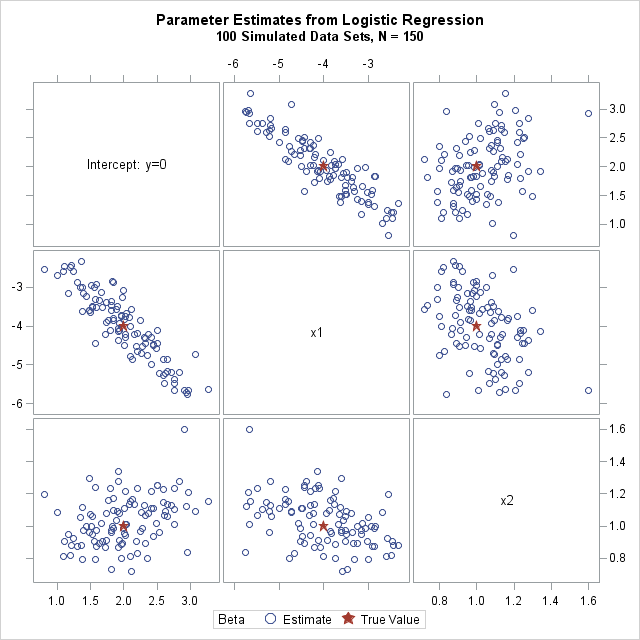The scatter plot matrix shows pairwise views of the sampling distribution of the three regression coefficients. The graph shows the variability of these estimates in a logistic model with the specified design matrix. It also shows how the coefficients are correlated.

By using the techniques in this post, you can simulate data for other generalized or mixed regression models. You can use similar techniques simulate data from other kinds of multivariate models.In my book Simulating Data with SAS, I show how to use the SAS DATA step to simulate data from a logistic regression model. Recently there have been discussions on the SAS/IML Support Community about simulating logistic data by using the SAS/IML language. This article describes how to efficiently simulate logistic data in SAS/IML, and is based on the DATA step example in my book.

The SAS documentation for the LOGISTIC procedure includes a brief discussion of the mathematics of logistic regression. To simulate logistic data, you need to do the following:

1. Assign the design matrix (X) of the explanatory variables. This step is done once. It establishes the values of the explanatory variables in the (simulated) study.
2. Compute the linear predictor, η = X β, where β is a vector of parameters. The parameters are the "true values" of the regression coefficients.
3. Transform the linear predictor by the logistic (inverse logit) function. The transformed values are in the range (0,1) and represent probabilities for each observation of the explanatory variables.
4. Simulate a binary response vector from the Bernoulli distribution, where each 0/1 response is randomly generated according to the specified probabilities from Step 3.

Presumably you are simulating the data so that you can call PROC LOGISTIC and obtain parameter estimates and other statistics for the simulated data. So, optionally, Step 5 is to write the data to a SAS data set so that PROC LOGISTIC can read it. Here's a SAS/IML program that generates a single data set of 150 simulated observations:

```/* Example from _Simulating Data with SAS_, p. 226--229 */ %let N = 150; /* N = sample size */ proc iml; call randseed(1); X = j(&N, 3, 1); /* X[,1] is intercept */ /* 1. Read design matrix for X or assign X randomly. For this example, x1 ~ U(0,1) and x2 ~ N(0,2) */ X[,2] = randfun(&N, "Uniform"); X[,3] = randfun(&N, "Normal", 0, 2);   /* Logistic model with parameters {2, -4, 1} */ beta = {2, -4, 1}; eta = X*beta; /* 2. linear model */ mu = logistic(eta); /* 3. transform by inverse logit */   /* 4. Simulate binary response. Notice that the "probability of success" is a vector (SAS/IML 12.1) */ y = j(&N,1); /* allocate response vector */ call randgen(y, "Bernoulli", mu); /* simulate binary response */   /* 5. Write y and x1-x2 to data set*/ varNames = "y" || ("x1":"x2"); Out = X; Out[,1] = y; /* simulated response in 1st column */ create LogisticData from Out[c=varNames]; /* no data is written yet */ append from Out; /* output this sample */ close LogisticData; quit;```

The SAS/IML statements for simulating logistic data are concise. A few comments on the program:

• The design matrix contains two continuous variables. In this simulation, one is uniformly distributed; the other is normally distributed. This was done for convenience. You could also read in a data set that defines the observed values of the explanatory variables. See the discussion and examples in Simulating Data with SAS, p. 201–205. (Notice also that I used the RANDFUN function in SAS/IML 12.3 to generate these variables.)
• The linear model does not contain an error term, as would be the case for linear regression. Instead, the binary response is random because we called the RANDGEN subroutine to generate a Bernoulli random variable.
• The parameter to the Bernoulli distribution is a vector of probabilities. This feature requires SAS/IML 12.1.
• So that the program can be extended to support an arbitrary number of explanatory variables, I copy all of the numerical data into the Out matrix, which is a copy of the X matrix except that the intercept column is replaced by the simulated binary responses. This matrix is written to a SAS data set.

What do the simulated data look like? The following call to SGPLOT produces a scatter plot of the X1 and X2 variables, with "events" colored red and "non-events" colored blue:

```proc sgplot data=LogisticData; scatter x=x1 y=x2 / group=y markerattrs=(symbol=CircleFilled); run;```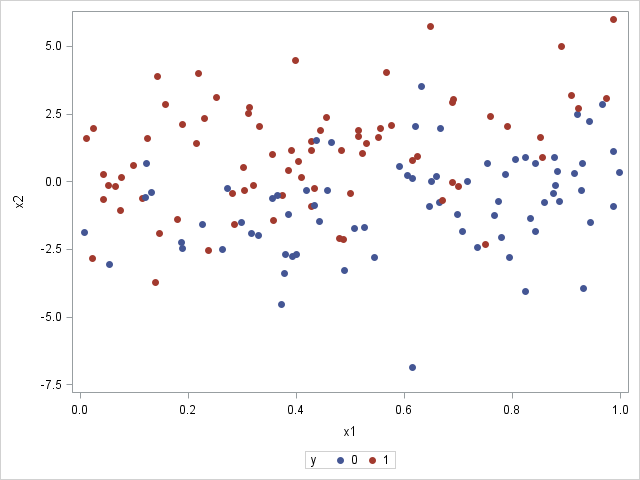The graph shows that the probability of an event decreases as you move from X1=0 (where there are many red markers) to X1=1 (where there are few red markers). This makes sense because the model coefficient for X1 is negative. In contrast, the probability of an event increases as you move from negative values of X2 to positive values. This makes sense because the model coefficient for X2 is positive. In general, most events (red markers) are in the upper left portion of the graph, whereas most non-events (blue markers) are in the lower right portion.

For small samples, there is a lot of uncertainty in the parameter estimates for a logistic regression. In this example, 150 observations were generated so that you can run PROC LOGISTIC against the simulated data and see that the parameter estimates are close to the parameter values. The following statement uses the CLMPARM=WALD option to compute parameter estimates and confidence intervals for the parameters:

```proc logistic data=LogisticData plots(only)=Effect; model y(Event='1') = x1 x2 / clparm=wald; ods select CLParmWald EffectPlot; run;```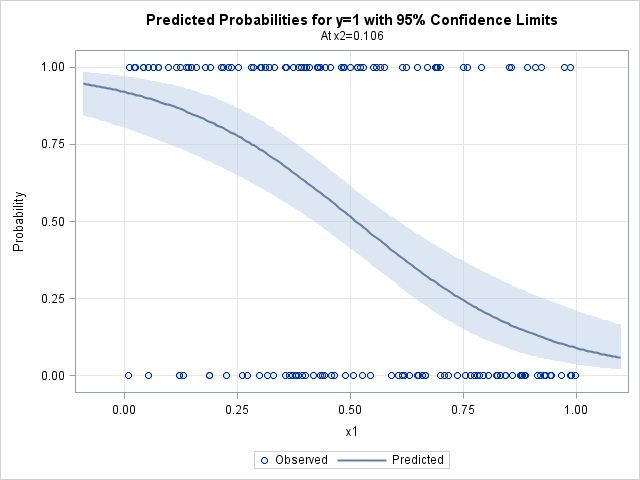Notice that the parameter estimates are somewhat close to the parameter values of (2, -4, 1). The 95% confidence intervals include the parameter values. (Re-run the program with N = 1000 or N = 10000 to see whether the estimates get closer to the parameters!) The graph shows the predicted probability of an event as a function of X1, conditioned on X2=0.1, which is the mean value of X2. You could request a similar plot for the predicted probability as a function of X2 (at the mean of X1) by using the option
plots(only)=Effect(showobs X=(X1 X2));

This article shows how to generate a single sample of data that fits a logistic regression model. In my next blog post, I will show you how to generalize this program to efficiently simulate and analyze many samples.A colleague asked me an interesting question:

I have a journal article that includes sample quantiles for a variable. Given a new data value, I want to approximate its quantile. I also want to simulate data from the distribution of the published data. Is that possible?

This situation is common. You want to run an algorithm on published data, but you don't have the data. Even if you attempt to contact the original investigators, the data are not always available. The investigators might be dead or retired, or the data are confidential or proprietary. If you cannot obtain the original data, one alternative is to simulate data based on the published descriptive statistics.

Simulating data based on descriptive statistics often requires making some assumptions about the distribution of the published data. My colleague had access to quantile data, such as might be produced by the UNIVARIATE procedure in SAS. To focus the discussion, let's look at an example. The table to the left shows some sample quantiles of the total cholesterol in 35 million men aged 40-59 in the years 1999–2002. The data were published as part of the NHANES study.

With 35 million observations, the empirical cumulative distribution function (ECDF) is a good approximation to the distribution of cholesterol in the entire male middle-aged population in the US. The published quantiles only give the ECDF at 11 points, but if you graph these quantiles and connect them with straight lines, you obtain an approximation to the empirical cumulative distribution function, as shown below:My colleague's first question is answered by looking at the plot. If a man's total cholesterol is 200, you can approximate the quantile by using linear interpolation between the known values. For 200, the quantile is about 0.41. If you want a more exact computation, you can use a linear interpolation program.

The same graph also suggest a way to simulate data based on published quantiles of the data. The graph shows an approximate ECDF by using interpolation to "connect the dots" of the quantiles. You can use the inverse CDF method for simulation to simulate data from this approximate ECDF. Here are the main steps for the simulation:

• Use interpolation (linear, cubic, or spline) to model the ECDF. For a large sample of a continuous variable, the approximate ECDF will be a monotonic increasing function from the possible range of the variable X onto the interval [0,1].
• Generate N random uniform values in [0,1]. Call these values u1, u2, ..., uN.
• Because the approximate ECDF is monotonic, for each value ui in [0,1] there is a unique value of x (call it xi) that is mapped onto ui. For cubic interpolation or spline interpolation, you need to use a root-finding algorithm to obtain each xi. For linear interpolation, the inverse mapping is also piecewise linear, so you can use a linear interpolation program to solve directly for xi.
• The N values x1, x2, ..., xN are distributed according to the approximate ECDF.

The following graph shows the distribution of 10,000 simulated values from the piecewise linear approximation of the ECDF. The following table compares the quantiles of the simulated data (the SimEst column) to the quantiles of the NHANES data. The quantiles are similar except in the tails. This is typical behavior because the extreme quantiles are more variable than the inner quantiles. You can download the SAS/IML program that simulates the data and that creates all the images in this article.A few comments on this method:

• The more quantiles there are, the better the ECDF is approximated by the function that interpolate the quantiles.
• Extreme quantiles are important for capturing the tail behavior.
• The simulated data will never exceed the range of the original data. In particular, the minimum and maximum value of the sample determine the range of the simulated data.
• Sometimes the minimum and maximum values of the data are not published. For example, the table might end begin with the 0.05 quantile and end with the 0.95 quantile. In this case, you have three choices:
• Assign missing values to the 10% of the simulated values that fall outside the published range. They simulated data will be from a truncated distribution of the data. The simulated quantiles will be biased.
• Use interpolation to estimate the minimum and maximum values of the data. For example, you could use the 0.90 and 0.95 quantiles to extrapolate a value for the maximum data value.
• Use domain-specific knowledge to supply reasonable values for the minimum and maximum data values. For example, if the data are positive, you could use 0 as a lower bound for the minimum data value.I've pointed out in the past that in the SAS/IML language matrices are passed to modules "by reference." This means that large matrices are not copied in and out of modules but are updated "in place." As a result, the SAS/IML language can be very efficient when it computes with large matrices. For example, when running large simulations, the SAS/IML language is often much faster than competing software that supports only function calls.

However, subroutines (which are called by using the CALL or RUN statements) are sometimes less convenient for a programmer to use. Each call to a subroutine is a separate statement. In contrast, you can nest function calls and use several function calls within a single complicated expression.

The convenience of functions is evident in some simulation studies. For example, you can regard the negative binomial distribution as a Poisson(λ) distribution, where λ is a gamma-distributed random variable. A distribution that has a random parameter is called a compound distribution. In a previous blog post I discussed the following program, which calls the RANDGEN subroutine to simulate data from a compound distribution:

```proc iml; call randseed(12345); N = 1000; a = 5; b = 2; /* Simulate X from a compound distribution: X ~ Poisson(lambda), where lambda ~ Gamma(a,b) */ lambda = j(N,1); call randgen(lambda, "Gamma", a, b); /* lambda ~ Gamma(a,b) */ x = j(N,1); call randgen(x, "Poisson", lambda); /* pass vector of parameters */```

After setting up the parameters, it takes four statements to simulate the data: two to allocate vectors to hold the results and two calls to the RANDGEN subroutine to fill those vectors with random values. Fewer statements are needed if a language supports a function that returns N random values.

Well, as of SAS/IML 12.3 (shipped with SAS 9.4), you can use the RANDFUN function when you want the convenience of calling a function that returns random values from a distribution. The syntax is
x = RANDFUN(N, "Distribution" <,param1> <,param2> <,param3>);
The following statements call the RANDFUN function to simulate data from a compound distribution:

```lambda = randfun(N, "Gamma", a, b); /* lambda ~ Gamma(a,b) */ x = randfun(N, "Poisson", lambda); /* X ~ Poisson(lambda) */```

If you want to nest the calls to the RANDFUN function, you can simulate the data with a single statement:

`x = randfun(N, "Poisson", randfun(N, "Gamma", a, b)); /* compound distribution */`

If you are simulating data inside a DO loop, I strongly suggest that you continue to use the more efficient RANDGEN subroutine. However, in applications where performance is not a factor, you can enjoy the convenience of simulating data with a function call by using the new RANDFUN function.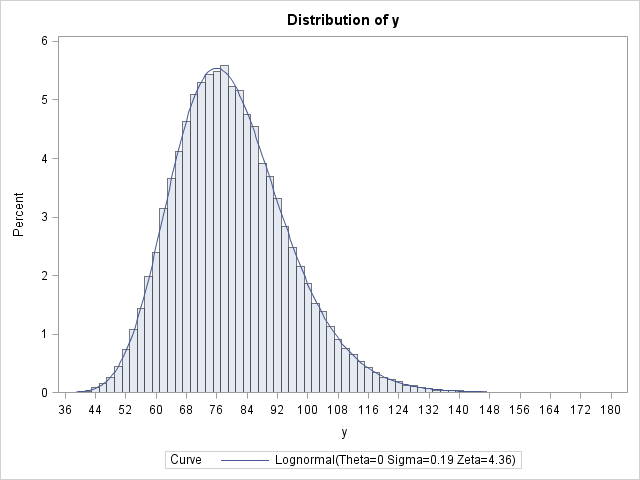In my book Simulating Data with SAS, I specify how to generate lognormal data with a shape and scale parameter. The method is simple: you use the RAND function to generate X ~ N(μ, σ), then compute Y = exp(X). The random variable Y is lognormally distributed with parameters μ and σ. This is the standard definition, but notice that the parameters are specified as the mean and standard deviation of X = log(Y).

Recently, a SAS customer asked me an interesting question. What if you know the mean and variance of Y, rather than log(Y)? Can you still simulate lognormal data from a distribution with that mean and variance?

Mathematically, the situation is that if m and v are the mean and variance, respectively, of a lognormally distributed variable Y, can you compute the usual parameters for log(Y)? The answer is yes. In terms of μ and σ, the mean of Y is m = exp(μ + σ2/2) and the variance is v = (exp(σ2) -1) exp(2μ + σ2). You can invert these formulas to get μ and σ as functions of m and v. Wikipedia includes these formulas in its article on the lognormal distribution, as follows: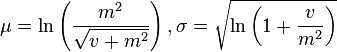Let's rewrite the expression inside logarithm. If you let φ = sqrt(v + m2), then the formulas are more simply written as
μ = ln(m2 / φ),     σ2 = ln(φ2 / m2 )
Consequently, you can specify the mean and the variance of the lognormal distribution of Y and derive the corresponding (usual) parameters for the underlying normal distribution of log(Y), as follows:

```data convert; m = 80; v = 225; /* mean and variance of Y */ phi = sqrt(v + m**2); mu = log(m**2/phi); /* mean of log(Y) */ sigma = sqrt(log(phi**2/m**2)); /* std dev of log(Y) */ run;   proc print noobs; run;```For completeness, let's simulate data from a lognormal distribution with a mean of 80 and a variance of 225 (that is, a standard deviation of 15). The previous computation enables you to find the parameters for the underlying normal distribution (μ and σ) and then exponentiate the simulated data:

```data lognormal; call streaminit(1); keep x y; m = 80; v = 225; /* specify mean and variance of Y */ phi = sqrt(v + m**2); mu = log(m**2/phi); sigma = sqrt(log(phi**2/m**2)); do i = 1 to 100000; x = rand('Normal', mu, sigma); y = exp(x); output; end; run;```

You can use the UNIVARIATE procedure to verify that the program was implemented correctly. The simulated data should have a sample mean that is close to 80 and a sample standard deviation that is close to 15. Furthermore, the LOGNORMAL option on the HISTOGRAM statement enables you to fit a lognormal distribution to the data. The fit should be good and the parameter estimates should be close to the parameter values μ = 4.36475 and σ = 0.18588 (except that PROC UNIVARIATE uses the Greek letter zeta instead of mu):

```ods select Moments Histogram ParameterEstimates; proc univariate data=lognormal; var y; histogram y / lognormal(zeta=EST sigma=EST); run;```

The histogram with fitted lognormal curve is shown at the top of this article. The mean of the simulated data is very close to 80 and the sample standard deviation is close to 15.My thanks to the SAS customer who asked this question—and researched most of the solution! It is a question that I had not previously considered.

Is this a good way to simulate lognormal data? It depends. If you have data and you want to simulate lognormal data that "looks just like it," I suggest that you run PROC UNIVARIATE on the real data and produce the maximum likelihood parameter estimates for the lognormal parameters μ and σ. You can then use those MLE estimates to simulate more data. However, sometimes the original data is not available. You might have only summary statistics that appear in some journal or textbook. In that case the approach in this article enables you to map the descriptive statistics of the original data to the lognormal parameters μ and σ so that you can simulate the unavailable data.While at a conference recently, I was asked whether it was possible to use SAS to simulate data from an inverse gamma distribution. The SAS customer had looked at the documentation for the RAND function and did not see "inverse gamma" listed among the possible choices.

The answer is "yes." The distributions that are listed for the RAND function (and the RANDGEN subroutine in SAS/IML) are building blocks that enable you to build other less common distributions. I discuss this in Chapter 7 of Simulating Data with SAS where I show how to simulate data from a dozen distributions that are not directly supported by the RAND function.

When I want to simulate data from a distribution that is not directly supported by the RAND function, I first look at the documentation for the MCMC procedure, which lists the definitions for more than 30 distributions. If I can't find the distribution there, I walk to the library to browse the ultimate reference guide: the two volumes of Continuous Univariate Distributions by Johnson, Kotz, and Balakrishnan (2nd edition, 1994). The Wikipedia article on the inverse gamma distribution uses the same parameterization as these sources, although that is not always the case. (In fact, the Wikipedia article includes a link to an alternative parameterization of the inverse gamma distribution as a scaled inverse chi-squared distribution.)

The reference sources indicate that it is trivial to generate data from the inverse gamma distribution. You first draw x from the gamma distribution with shape parameter a and scale 1/b. Then the reciprocal 1/x is a draw from the inverse gamma distribution with shape parameter a and scale b. You can do this in the DATA step by using the RAND function, or in the SAS/IML language by using the following program:

```proc iml; call randseed(1); a= 3; /* shape parameter */ b= 0.5; /* scale for inverse gamma */ x = j(1000,1); /* 1000 draws */ call randgen(x, "Gamma", a, 1/b); /* X ~ gamma(a,1/b) */ x = 1/x; /* reciprocal is inverse gamma */```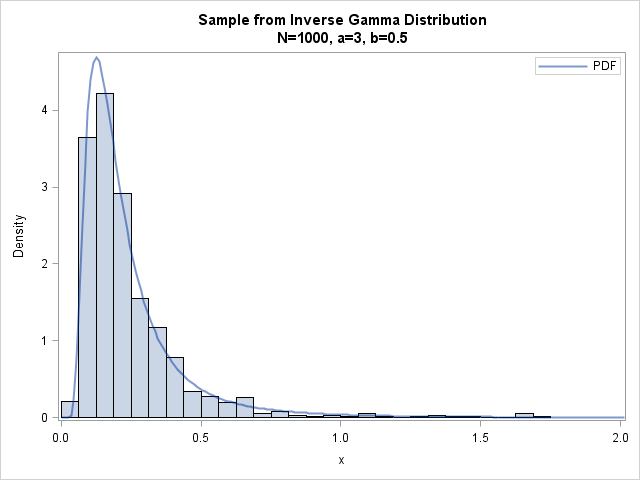The histogram to the left shows the distribution of 1000 draws from the inverse gamma distribution with parameters a=3 and b=0.5. The PDF of the inverse gamma distribution is overlaid on the histogram. (For details of this technique, see the article "How to overlay a custom density on a histogram in SAS.") The simulated data extend out past x=5, but the histogram and PDF have been truncated at x=2 to better show the density near the mode b/(1+a) = 1/8.

The lesson to learn is that it is often straightforward to use the elementary distributions in SAS to construct more complicated distributions. There are infinitely many distributions, so no software documentation can ever explicitly list them all! However, you can often use elementary arithmetic operations to express a complicated distribution in terms of simpler distributions.I began 2014 by compiling a list of 13 popular articles from my blog in 2013. Although this "People's Choice" list contains many articles that I am proud of, it did not include all of my favorites, so I decided to compile an "Editor's Choice" list. The blog posts on today's list were not widely popular, but they deserve a second look because they describe SAS computations that are elegant, surprising, or just plain useful.

I often write about four broad topics: the SAS/IML language, statistical programming, simulating data, and data analysis and visualization. My previous article included five articles on statistical graphics and data analysis, so here are a few of my favorite articles from the other categories.

### The SAS/IML language and matrix programming

Here are a few articles that can help you get more out of the SAS/IML product:

Serious SAS/IML programmers should read my book Statistical Programming with SAS/IML Software.

### Simulating data with SAS

Here are a few of my favorite articles from 2013 about efficient simulation of data:

Readers who want to learn more about simulating data might enjoy my book Simulating Data with SAS, which contains hundreds of examples and exercises.

### Statistical programming and computing

Much of my formal training is in numerical analysis and matrix computations. Here are a few interesting computational articles that I wrote. Be warned: some of these articles have a high "geek factor"!

There you have it, my choice of 12 articles that I think are worth a second look. What is your favorite post from The DO Loop in 2013? Leave a comment.My previous post described the multinomial distribution and showed how to generate random data from the multinomial distribution in SAS by using the RANDMULTINOMIAL function in SAS/IML software. The RANDMULTINOMIAL function is simple to use and implements an efficient algorithm called the sequential conditional marginal method (see Gentle (2003), p. 198–199). This algorithm is efficient even when the N parameter in the multinomial distribution is large.

If you do not have SAS/IML software, you can use the DATA step to simulate multinomial data in SAS. The most straightforward way is to simulate N draws from k categorical values and count the number of draws in each category. This is not as efficient as the algorithm that the RANDMULTINOMIAL function uses, but you can use this alternative method when N is small.

The following DATA step uses the "Table" distribution to generate categorical data according to specified probabilities and counts the number of each category:

```%let SampleSize = 1000; %let NumTrials = 100;   /* Generate multinomial data when NumTrials is not large */ data multinomial(drop=i j); array probs{3} _temporary_ (0.5 0.2 0.3); /* prob of i_th category */ array x{3} x1-x3; /* store counts */ call streaminit(1234); do Replicate = 1 to &SampleSize; do i = 1 to dim(x); x[i] = 0; end; /* zero the counts */ do i = 1 to &NumTrials; j = rand("table", of probs[*]); /* category 1-k */ x[j]+1; /* increment count */ end; output; end; run;   proc print data=multinomial(obs=10) noobs; var Replicate x:; run;```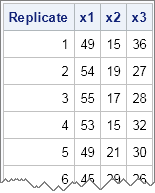In the DATA step, the RAND function generates a random number in the range 1–k, where k = 3 for this example. An array keeps track of the count of each category. Each row of the resulting data set contains one observation from the multinomial distribution. Each row sums to &NumTrials, which is 100 for this example.

Each row can be interpreted as the result of drawing 100 items (with replacement) from a box that contains items of three colors. The first row is interpreted as drawing 49 items of the first color, 15 items of the second color, and 36 items of the third color. The second row represents a second independent draw of 100 items.

I've seen many variations on this DATA step technique over the years, but recently I saw a blog post by Simon Huang that uses the SURVEYSELECT procedure instead of the DATA step. The idea is to specify the vector of probabilities in a data set and then use PPS (probability proportional to size) sampling to sample with replacement. The SIZE statement specifies the variable in the data set that contains the sampling probabilities, as follows:

```/* define a data set that contains the categories and probabilities */ data Probs; array probs{3} _temporary_ (0.5 0.2 0.3); do x = 1 to dim(probs); prob = probs{x}; output; end;   %let SampleSize = 1000; %let NumTrials = 100; proc surveyselect data=Probs noprint seed=1234 method=pps_wr out=sample(drop=prob ExpectedHits SamplingWeight) reps = &SampleSize /* number of observations from multinomial distribution */ N = &NumTrials; /* number of trials */ id x; size prob; run;```

The SURVEYSELECT procedure creates an output data set in "long" form. The data set contains three variables: the variable Replicate contains the values 1–1000, the variable x contains the categories 1,2,...,k, and the variable NumberHits contains the number of times that category i was drawn out of N trials.

You can use PROC TRANSPOSE to convert the data set from long to wide form, as follows:

```/* long to wide transpose */ proc transpose data=sample out=multinomial prefix=x; by Replicate; id x; var NumberHits; run;   proc print data=multinomial(obs=10) noobs; var Replicate x:; run;```

The resulting data set is identical to the data set that is created by the DATA step, provided that no category has zero counts. If a category for some trial has zero counts, the TRANSPOSE procedure will insert a missing value instead of a zero. (To see this, use %let NumTrials = 100;) If your application requires a zero instead of a missing value, use a subsequent DATA step (not shown) to convert the missing values to zeros, or just use the DATA step program at the beginning of this article.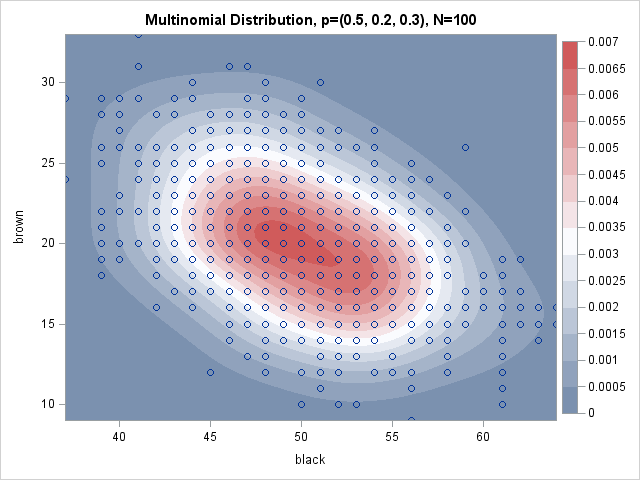This article describes how to generate random samples from the multinomial distribution in SAS. The content is taken from Chapter 8 of my book Simulating Data with SAS.

The multinomial distribution is a discrete multivariate distribution. Suppose there are k different types of items in a box, such as a box of marbles with k different colors. Let pi be the probability of drawing an item of type i, where Σi pi = 1. If you draw N items with replacement, you will obtain N1 items of the first type, N2 items of the second type, and so forth, where Σi Ni = N. The k-tuple of counts (N1, N2, ..., Nk) is a single draw from the multinomial distribution.

For example, suppose you want to draw socks (with replacement) from a big box that contains socks of k = 3 different colors. After each draw, you record the color of the sock, replace it in the box, and mix the contents well so that the probabilities of drawing each color are unchanged. If you draw N = 100 socks, you might get 48 black socks, 21 brown socks, and 31 white socks. That triplet of values, (48, 21, 31), is a single observation for the multinomial distribution of three categories (the colors). Of course, repeating the experiment will, in general, result is a different triplet of counts. The distribution of those counts is the multinomial distribution. The scatter plot at the top of this article visualizes the distribution for the parameters p = (0.5, 0.2, 0.3) and for N = 100. Notice that only two counts are shown; the third count is 100 minus the sum of the first two counts.

Notice that if there are only two categories with selection probabilities, p and 1 – p, then the multinomial distribution is equivalent to the binomial distribution with parameter p in the sense that the count N1 is binomially distributed (and so is N2).

You can sample from the multinomial distribution in SAS by using the RANDMULTINOMIAL function in SAS/IML software. The syntax of the RANDMULTINOMIAL function is

` X = RandMultinomial(SampleSize, NumTrials, Prob);`
where SampleSize is the requested number of observations to draw, NumTrials is the number of independent draws of the k categories, and Prob is a 1 x k vector of probabilities that sum to 1.

The following statements generate a 1000 x 3 matrix, where each row is a random observation from a multinomial distribution. The probability of drawing a black sock is 0.5. The probability of drawing a brown sock is 0.2. The probability of drawing a white sock is 0.3.

```proc iml; call randseed(4321); /* set random number seed */ c = {"black", "brown", "white"}; prob = {0.5 0.2 0.3}; /* probabilities of pulling each color */ X = RandMultinomial(1000, 100, prob); /* 1000 draws of 100 socks with 3 colors */ print X[colname=c];```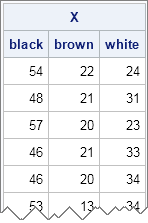Each row shows the frequencies that result from drawing 100 socks (with replacement). Consequently, the sum of each row is 100. Although there is variation within each column, elements in the first column are usually close to the expected value of 50, elements in the second column are close to 20, and elements in the third column are close to 30. You can see this by computing the column means. You can also compute the correlation matrix, which shows that the variables are negatively correlated with each other. This makes sense: There are exactly 100 items in each draw, so if you draw more of one color, you will have fewer of the other colors.

```mean = mean(X); corr = corr(X); print mean[colname=c], corr[colname=c rowname=c format=BEST5.];```You can visualize the multinomial distribution by plotting the first two components against each other, as shown at the beginning of this post. The multinomial distribution is a discrete distribution whose values are counts, so there is considerable overplotting in a scatter plot of the counts. One way to resolve the overplotting is to overlay a kernel density estimate. Areas of high density correspond to areas where there are many overlapping points.

```create MN from X[c=c]; append from X; close MN; quit;   ods graphics on; proc kde data=MN; bivar black brown / bwm=1.5 plots=ContourScatter; run;```

The density overlay shows that most of the density is near the population mean (50, 20). The scatter plot overlay shows the negative correlation in the simulated data, and also that some observations are far from the mean.

If you don't have SAS/IML software, you can still simulate multinomial data by using the "table distribution" in the data step. There is also a clever way to use PROC SURVEYSELECT to generate multinomial data. I'll describe those alternate computations in my next blog post.